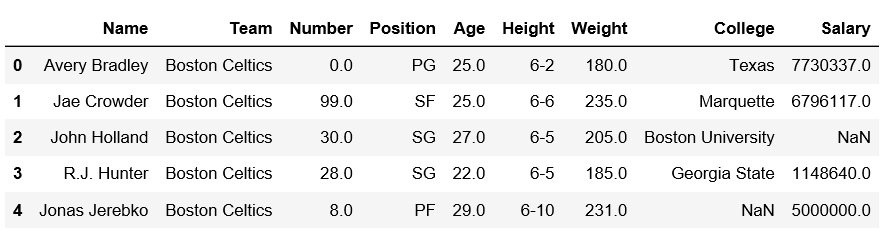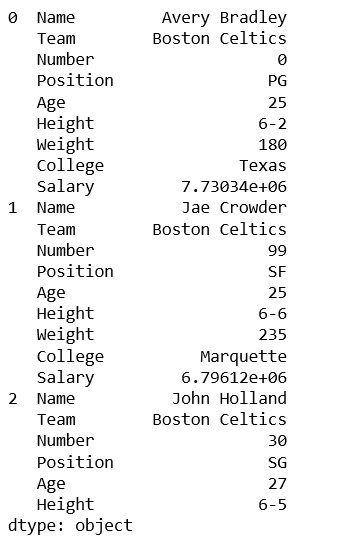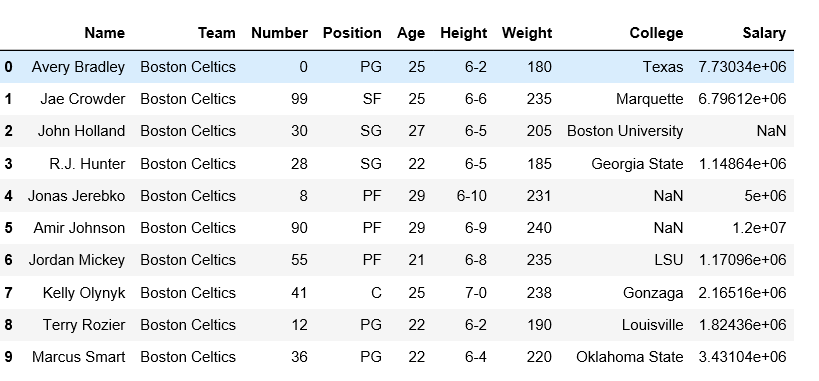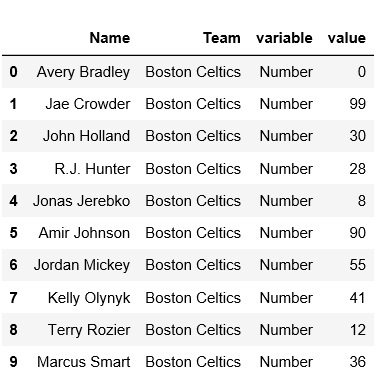Skip to content
Related Articles
Reshape a pandas DataFrame using stack,unstack and melt method
• Last Updated : 08 Jan, 2019

Pandas use various methods to reshape the dataframe and series. Let’s see about the some of that reshaping method.

Let’s import a dataframe first.

 `# import pandas module``import` `pandas as pd`` ` `# making dataframe``df ``=` `pd.read_csv(``"https://media.geeksforgeeks.org/wp-content/uploads/nba.csv"``)`` ` `# it was print the first 5-rows``print``(df.head()) `

Output:Using stack() method:

Stack method works with the MultiIndex objects in DataFrame, it returning a DataFrame with an index with a new inner-most level of row labels. It changes the wide table to a long table.

 `# import pandas module``import` `pandas as pd`` ` `# making dataframe``df ``=` `pd.read_csv(``"nba.csv"``)`` ` `# reshape the dataframe using stack() method``df_stacked ``=` `df.stack()`` ` `print``(df_stacked.head(``26``))`

Output:Using unstack() method:
`unstack `is similar to stack method, It also works with multi-index objects in dataframe, producing a reshaped DataFrame with a new inner-most level of column labels.

 `# import pandas module``import` `pandas as pd`` ` `# making dataframe``df ``=` `pd.read_csv(``"nba.csv"``)`` ` `# unstack() method``df_unstacked ``=` `df_stacked.unstack()``print``(df_unstacked.head(``10``))`Using `melt()` method:
Melt in pandas reshape dataframe from wide format to long format. It uses the “id_vars[‘col_names’]” for melt the dataframe by column names.

 `# import pandas module``import` `pandas as pd`` ` `# making dataframe``df ``=` `pd.read_csv(``"nba.csv"``)`` ` `# it takes two columns "Name" and "Team"``df_melt ``=` `df.melt(id_vars ``=``[``'Name'``, ``'Team'``]) ``print``(df_melt.head(``10``))`

Output:Attention geek! Strengthen your foundations with the Python Programming Foundation Course and learn the basics.

To begin with, your interview preparations Enhance your Data Structures concepts with the Python DS Course. And to begin with your Machine Learning Journey, join the Machine Learning – Basic Level Course

My Personal Notes arrow_drop_up